# How to Implement a Binary Search Tree

A binary search tree, or BST, is a type of data structure typically used to organize data. It is structured in a very different way compared to stacks, queues and lists. Generally, a BST will have the following properties.

1. The tree has a root node that is used as the starting point for any operation

2. Each node in the tree has one or two nodes attached to it. One is to the right of the node, and the other is to the left.

3. Everything to the right of a node is larger in value compared to it. Everything to the left of a node is smaller in value compared to it.

The main valuable property of BSTs is that they store data in a way that is easy to search. When data is stored in a BST, we can use the property of left and right nodes to determine where a node we are searching for is likely to be. In general, it takes less time to search a BST compared to searching through a list of values one by one.

To start implementing a tree, we first need to create a new type of node that has a left and right property. This node object isn’t too different from the node objects used for lists. The only difference is that the new node object has two links instead of one. It still has a value, and still implements the same getters and setters to manipulate its values.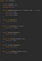A TreeNode implementation

Once we have a Treenode implemented, we can use it to create a BST object. The two main operations we want to implement for our BST object is an operation to insert values, and an operation to remove values. Once we have these two operations, we can easily extend the structures to add more later.

Our BST object will have just a single property, which is the root of the tree itself. The root will be able to be iterated at any time to determine any other values within the tree. The insert method is implemented using recursion, which will be the case for almost every tree method. To get a better idea of what we need to do for our insert method, let’s look at an example.

Suppose we wanted to insert the values [5,2,7,6,4,3] into our BST, in the order given. When we start, the BST will be empty, meaning that we just need to update the root with the value 5.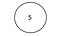We start with a single root node

The next value after this is 2. To insert this, we need to check the root to see if we are less than or greater than its value. Since 2 is less than 5, it will go the left of 5. There are no other values in the tree, so we just update the roots left pointer and we are done. On the other hand, 7 is greater than 5, so it goes to the right of 5. Since there are no other values to the right, we update the root right pointer to 5 and we are done.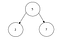Inserts go to the left and right of the root

The insert starts to get more complicated once the left and right of the root are filled in. The next element we want to insert is 6. This is greater than the root, so we go to the right of it and reach 7. From here, we need to do another comparison to see that 6 is less than 7. We then update the left of 7 to be 6. You can see from this that we need to keep comparing until we reach an empty spot. Once we reach an empty spot, we insert there, and we are done. If we continue with this pattern, we get the tree below.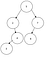The final tree after all inserts

From this example, we can see that our stopping condition is that the node we are currently at is null. We make recursive calls on cases where the value we are inserting is less than or greater than the current one. If we encounter a situation where we are equal to the value we are inserting, we don’t insert the value since it already exists. With BSTs, we typically use a convention that duplicate values are not allowed. Using this logic, we can construct a recursive function that will insert a value into our BST.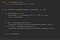Our insert method implementation

This code follows the general format of what we discussed in the example. If the current node we are at is not null, we move down the left or right of the tree, updating on each recursive call. Once we reach a null value, or a value that is equal to what we wish to insert, we move up the recursive stack, updating as we go, ending with a fully updated tree with the new node inserted.

Now that we have an insert, the idea of removing from the tree follows similarly in logic. We need to first locate the value we wish to remove inside of the tree. We do this by starting at the root and moving left or right depending on if the current value is smaller or larger than the value we want. Once we find the value, we remove it from the tree, updating all the previous nodes through recursion. Doing so will give us an updated tree with the targeted node removed.

The actual process of removing the node once we find it is a little trickier. We need to locate an adequate replacement from the children of the node we are removing. Let’s look at the code to see how this is done.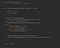The remove method

The first part of this code is like our insert method. We move to the left and right in the tree searching for the value we want to remove. The new logic comes in when we find the value we wish to remove. In the case where the node has only one value linked to it, the remove is easy. We simply replace with the value that exists, either the left or right.

If there is both a left and right, then we need to find the smallest node that exists to the right of the node we want to remove. Once we do this, we recursively call the function to update the right-hand side to link based on the smallest value that succeeds the one being removed. We find the smallest value by iterating the left-hand side of the node we are removing, since everything to the left is smaller than what came before it.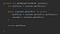The min value method

Doing this gives us the full functionality required to remove nodes from a tree. The insert and remove methods provide the fundamental ideas required to traverse a tree, and update values when required. Using these concepts, you will be able to implement any tree related recursion, since most of the methods will follow a similar idea of iterating the tree to find or update values.

Computer programmer specializing in security. My blog: www.scprogramming.com

## More from Scott Cosentino

Computer programmer specializing in security. My blog: www.scprogramming.com